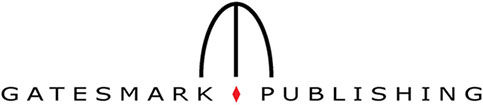| Home | Contact us | Search |

 DIPUM Toolbox Version 2 Function List
 Function Description Image Display and Exploration ice Interactive Color Editor. rgbcube Displays an RGB cube on the MATLAB desktop. Image file I/O movie2tifs Creates a multiframe TIFF file from a MATLAB movie. seq2tifs (DIPUM) Creates a multi-frame TIFF file from a MATLAB sequence. tifs2movie Create a MATLAB movie from a multiframe TIFF file. tifs2seq Create a MATLAB sequence from a multi-frame TIFF file. Geometric Transformations imtransform2 2-D image transformation with fixed output location. pixeldup Duplicates pixels of an image in both directions. pointgrid Points arranged on a grid. reprotate Rotate image repeatedly. vistform Visualization transformation effect on set of points. Image Registration visreg Visualize registered images. Pixel Values and Statistics localmean Computes an array of local means. statmoments Computes statistical central moments of image histogram. Image Analysis bayesgauss Bayes classifier for Gaussian patterns. bound2eight Convert 4-connected boundary to 8-connected boundary. bound2four Convert 8-connected boundary to 4-connected boundary. bound2im Converts a boundary to an image. bsubsamp Subsample a boundary. bwboundaries Trace region boundaries in binary image. colorgrad Computes the vector gradient of an RGB image. colorseg Performs segmentation of a color image. connectpoly Connects vertices of a polygon. cornerprocess Processes the output of function cornermetric. diameter Measure diameter and related properties of image regions. fchcode Computes the Freeman chain code of a boundary. frdescp Computes Fourier descriptors. ifrdescp Computes inverse Fourier descriptors. im2minperpoly Minimum perimeter polygon. imstack2vectors Extracts vectors from an image stack. invmoments Compute invariant moments of image. localthresh Local thresholding. mahalanobis Computes the Mahalanobis distance. movingthresh Image segmentation using a moving average threshold. otsuthresh Otsu's optimum threshold given a histogram. polyangles Computes internal polygon angles. principalcomps Principal-component vectors and related quantities. randvertex Adds random noise to the vertices of a polygon. regiongrow Perform segmentation by region growing. signature Computes the signature of a boundary. specxture Computes spectral texture of an image. splitmerge Segment an image using a split-and-merge algorithm. statxture Computes statistical measures of texture in an image. strsimilarity Computes a similarity measure between two strings. x2majoraxis Aligns coordinate x with the major axis of a region. Image Compression compare Computes and displays the error between two matrices. cv2tifs Decodes a TIFS2CV compressed image sequence. huff2mat Decodes a Huffman encoded matrix. huffman Builds a variable-length Huffman code for a symbol source. im2jpeg Compresses an image using a JPEG approximation. im2jpeg2k Compresses an image using a JPEG 2000 approximation. imratio Computes the ratio of the bytes in two images/variables. jpeg2im Decodes an IM2JPEG compressed image. jpeg2k2im Decodes an IM2JPEG2K compressed image. lpc2mat Decompresses a 1-D lossless predictive encoded matrix. mat2huff Huffman encodes a matrix. mat2lpc Compresses a matrix using 1-D lossles predictive coding. ntrop Computes a first-order estimate of the entropy of a matrix. quantize Quantizes the elements of a UINT8 matrix. showmo Displays the motion vectors of a compressed image sequence. tifs2cv Compresses a multi-frame TIFF image sequence. unravel Decodes a variable-length bit stream. Image Enhancement adpmedian Perform adaptive median filtering. gscale Scales the intensity of the input image. intrans Performs intensity (gray-level) transformations. Image Noise imnoise2 Generates an array of random numbers with a specified PDF. imnoise3 Generates periodic noise. Linear Filtering dftfilt Performs frequency domain filtering. spfilt Performs linear and nonlinear spatial filtering. Linear 2-D Filter Design bandfilter Computes frequency domain band filters. cnotch Generates circularly symmetric notch filters. hpfilter Computes frequency domain highpass filters lpfilter Computes frequency domain lowpass filters. recnotch Generates rectangular notch (axes) filters. Fuzzy Logic aggfcn Aggregation function for a fuzzy system. approxfcn Approximation function. bellmf Bell-shaped membership function. defuzzify Output of fuzzy system. fuzzyfilt Fuzzy edge detector. fuzzysysfcn Fuzzy system function. implfcns Implication functions for a fuzzy system. lambdafcns Lambda functions for a set of fuzzy rules. makefuzzyedgesys Script to make MAT-file used by FUZZYFILT. onemf Constant membership function (one). sigmamf Sigma membership function. smf S-shaped membership function. trapezmf Trapezoidal membership function. triangmf Triangular membership function. truncgaussmf Truncated Gaussian membership function. zeromf Constant membership function (zero). Morphological Operations (Binary Images) endpoints Computes end points of a binary image. Texture Analysis specxture Computes spectral texture of an image. statxture Computes statistical measures of texture in an image. Region-Based Processing histroi Computes the histogram of an ROI in an image. Wavelets waveback Performs a multi-level, 2-dimensional inverse FWT. wavecopy Fetches coefficients of a wavelet decomposition structure. wavecut Sets to zero coefficients in a wavelet decomposition structure. wavedisplay Display wavelet decomposition coefficients. wavefast Performs a multilevel 2-dimensional fast wavelet transform. wavefilter Creates wavelet decomposition and reconstruction filters. wavepaste Puts coefficients in a wavelet decomposition structure. wavework Edits wavelet decomposition structures. wavezero Sets wavelet detail coefficients to zero. Color Space Conversions hsi2rgb Converts HSI values to RGB color space. rgb2hsi Converts RGB values to HSI color space. Array Operations dftuv Computes meshgrid arrays. paddedsize Computes the minimum required pad size for use in FFTs. Image Types and Type Conversions tofloat Convert image to floating point. Miscellaneous Functions conwaylaws Applies Conway’s genetic laws to a single pixel. i2percentile Computes a percentile given an intensity value. iseven Determines which elements of an array are even numbers. isodd Determines which elements of an array are odd numbers. manualhist Generates a bimodal histogram interactively. percentile2i Computes an intensity value given a percentile. timeit Measure time required to run function. twomodegauss Generates a bimodal Gaussian function.